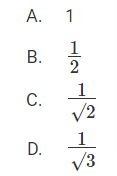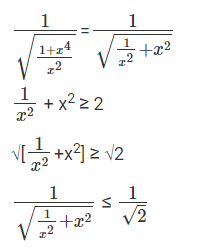# CAT Prep 2022 | CAT Previous Year Question Papers With Solution | CAT 2022 Question Bank | PaGaLGuY

CAT 2020 Quant Question: Geometry

From the interior point of an equilateral triangle, perpendiculars are drawn on all three sides. The sum of the lengths of the perpendiculars is ‘s’. Then the area of the triangle is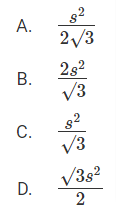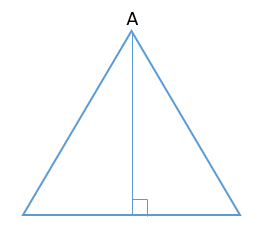From any point on a equilateral triangle, if we draw three perpendiculars on other sides
Those three perpendicular will add up to = Altitude of an equilateral triangle
We take the point to be A, and try to draw 3 perpendiculars (Can draw only one)
And is same as altitude of the equilateral triangle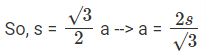By substituting the value in Area of an equilateral triangle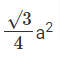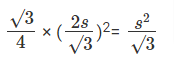CAT 2020 Quant Question: Averages

In a group of 10 students, the mean of the lowest 9 scores is 42 while the mean of the highest 9 scores is 47. For the entire group of 10 students, the maximum possible mean exceeds the minimum possible mean by

A. 5
B. 3
C. 4
D. 6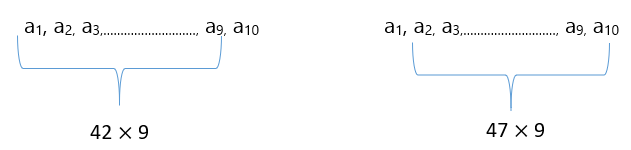Here a2 to a9 is common to both the terms
So, a1 + (a2 to a9) = 42 × 9
a10 + (a2 to a9) = 47 × 9
Solving these two a10 - a1 = 45
a1, a2, a3,………………………, a9, (a1 + 45)
One instance is every number is 42
42, 42, …………………, 42, 42+45 (a1 to a9 are equal) -----------(1)
Another instance is every number is 47
47 – 45, 47, …………………, 47, 47 (a2 to a10 are equal) ---------(2)
Mean of (1) = 46.5
Mean of (2) = 42.5
(1) – (2) = 4

CAT 2020 Quant Question: Linear & quadratic equations

The number of pairs of integers(x,y) satisfying x ≥ y ≥ -20 and 2x + 5y = 99 is

x = 2 and y = 19 (Not possible), Given the condition x ≥ y ≥ - 20
x should increase/decrease in the coefficient of y and same for y
x = 7 and y = 17 (Not possible)
x = 12 and y = 15 (Not possible)
x = 17 and y = 13 (Possible)
. y = 11
. y = 9
. .
. .
. .
. y = -19 (Can’t go any further)
By counting totally 17 values are there

Hence, the answer is, " 17"

CAT 2020 Quant Question: Logarithms

The value of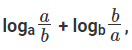for 1 < a ≤ b cannot be equal to

A. -0.5
B. 1
C. 0
D. -1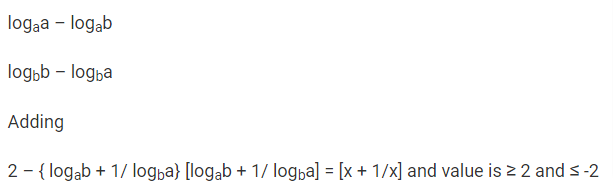From the options,
x + 1/x ≠ 1

CAT 2020 Quant Question: Sequence & series

Let teh m-th and n-thterms of a Geometric progression be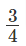and 12, respectively, when m < n. If the common ratio of the progression is an integer r, then the smallest possible value of r + n - m is

A. -4
B. -2
C. 6
D. 2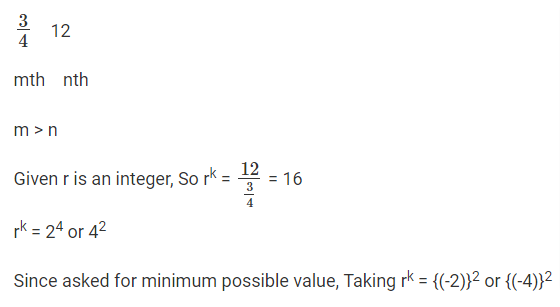k = n – m (From m how many terms we have to jump to reach n)
We have two cases r = - 2 and n - m = 4 ----> r + n – m = 2
r = - 4 and n – m = 2 ----> r + n – m = - 2
-2 is the smallest possible value

CAT 2020 Quant Question: Simple interest & Compound interest

For the same principal amount, the compound interest for two years at 5% per annum exceeds the simple interest for three years at 3% per annum by Rs 1125. Then the principal amount in rupees is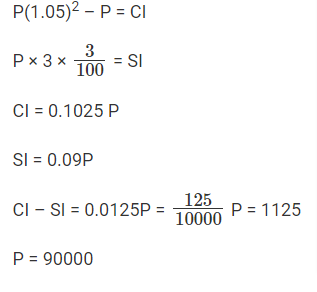Hence, the answer is, " 90000"

CAT 2020 Quant Question: Geometry

Let C be a circle of radius 5 meters having center at O. Let PQ be a chord of C that passes through points A and B where A is located 4 meters north of O and B is located 3 meters east of O. Then, the length of PQ, in meters, is nearest to

A. 6.6
B. 7.2
C. 8.8
D. 7.8O is the center of the circle and radius of circle = 5
In triangle AOB, using pythagorean triplet AB = 5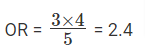Since OR if from the center it bisects the chord,
PR = RQ
Now in triangle POR,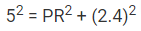PR = √{19.24}
PQ = 2 √{19.24}
PQ ≅ 8.8

For real x, the maximum possible value of